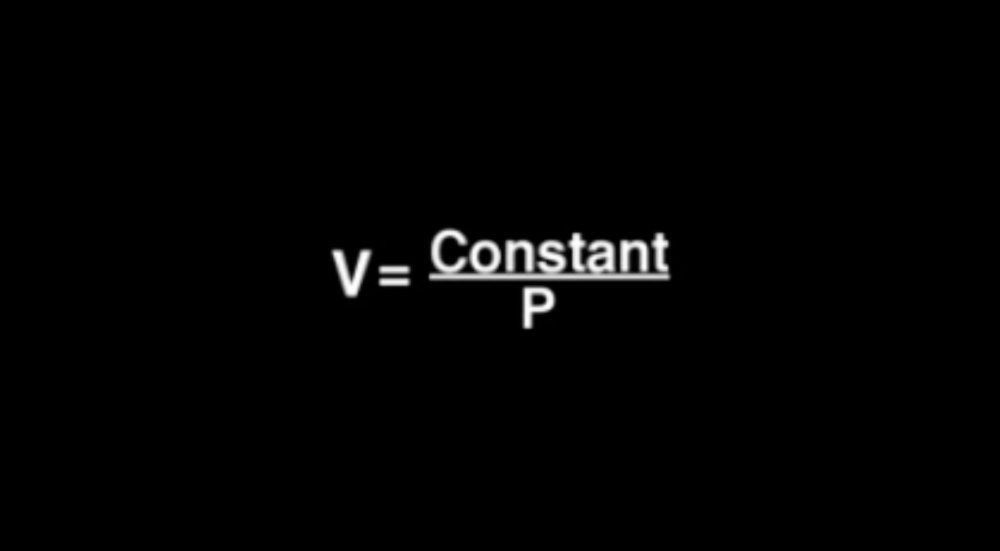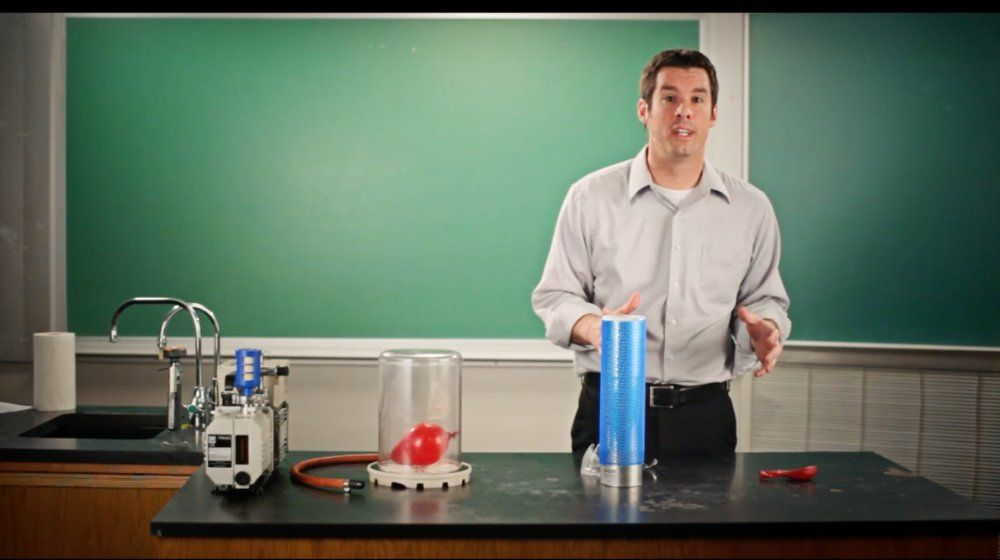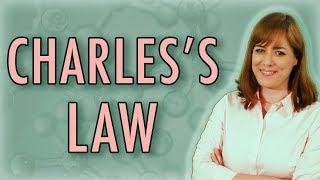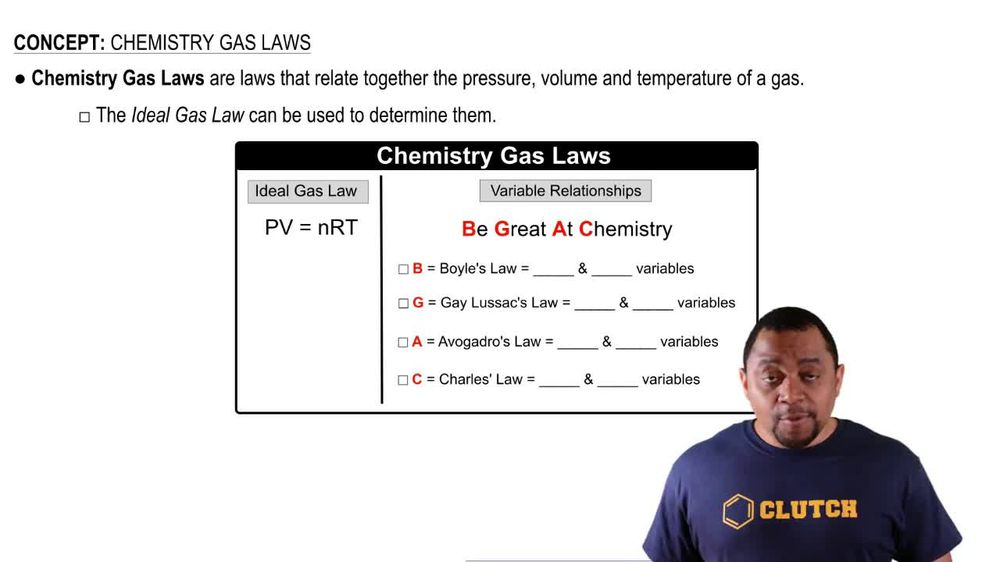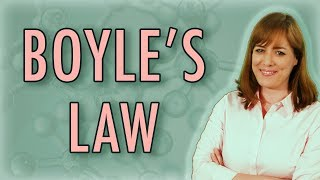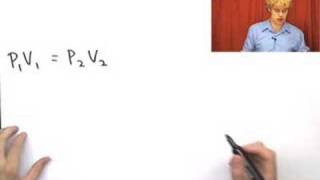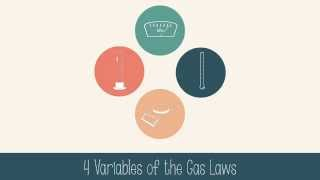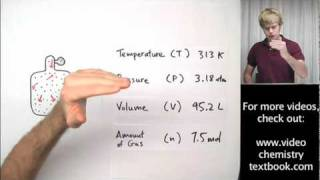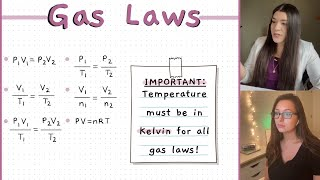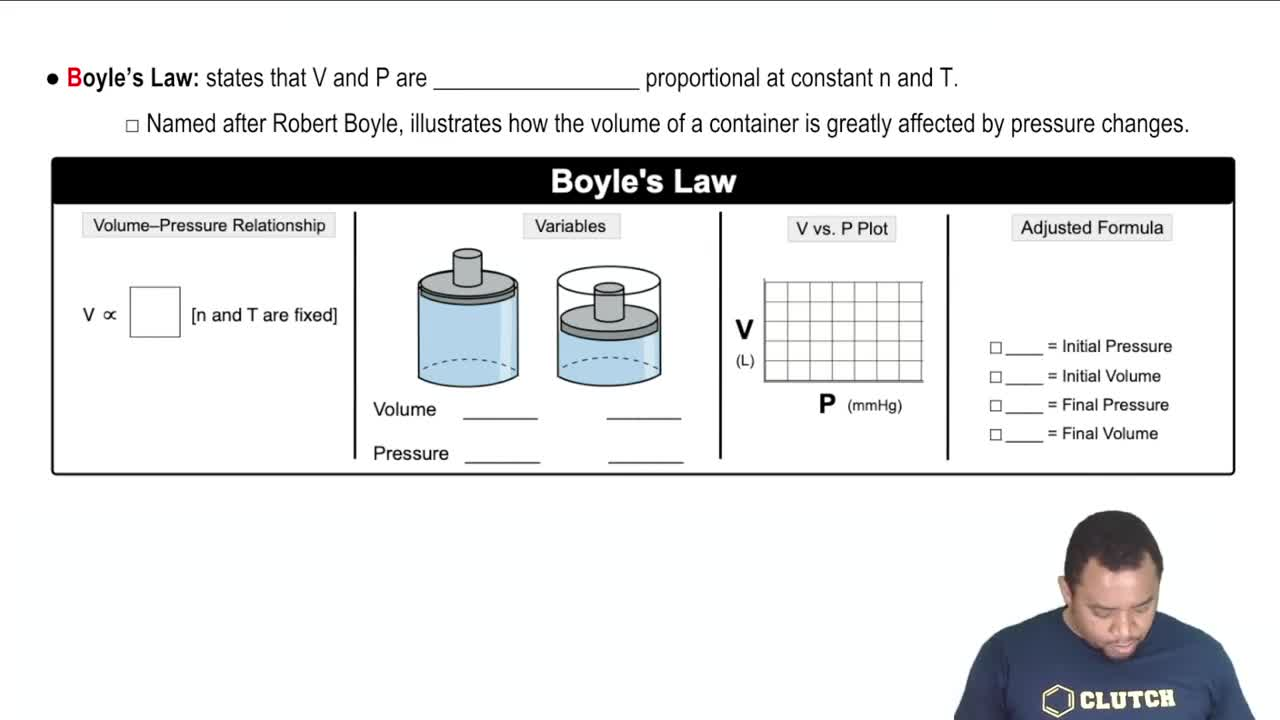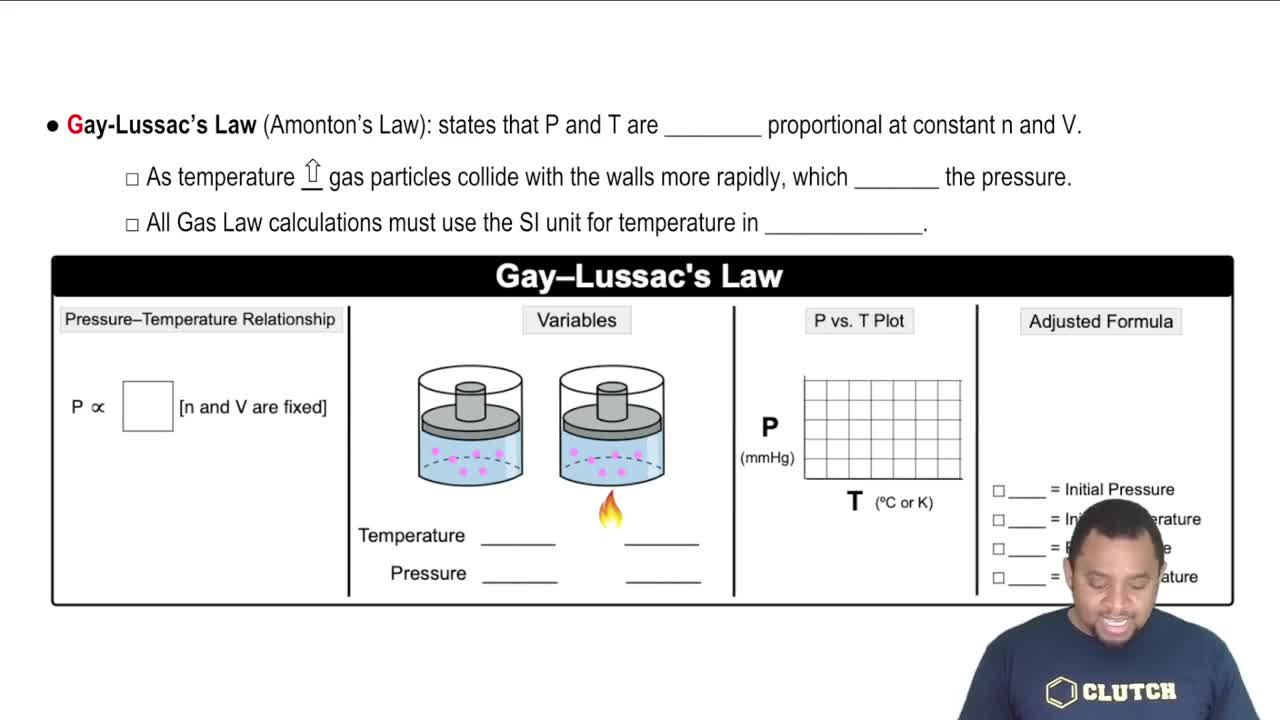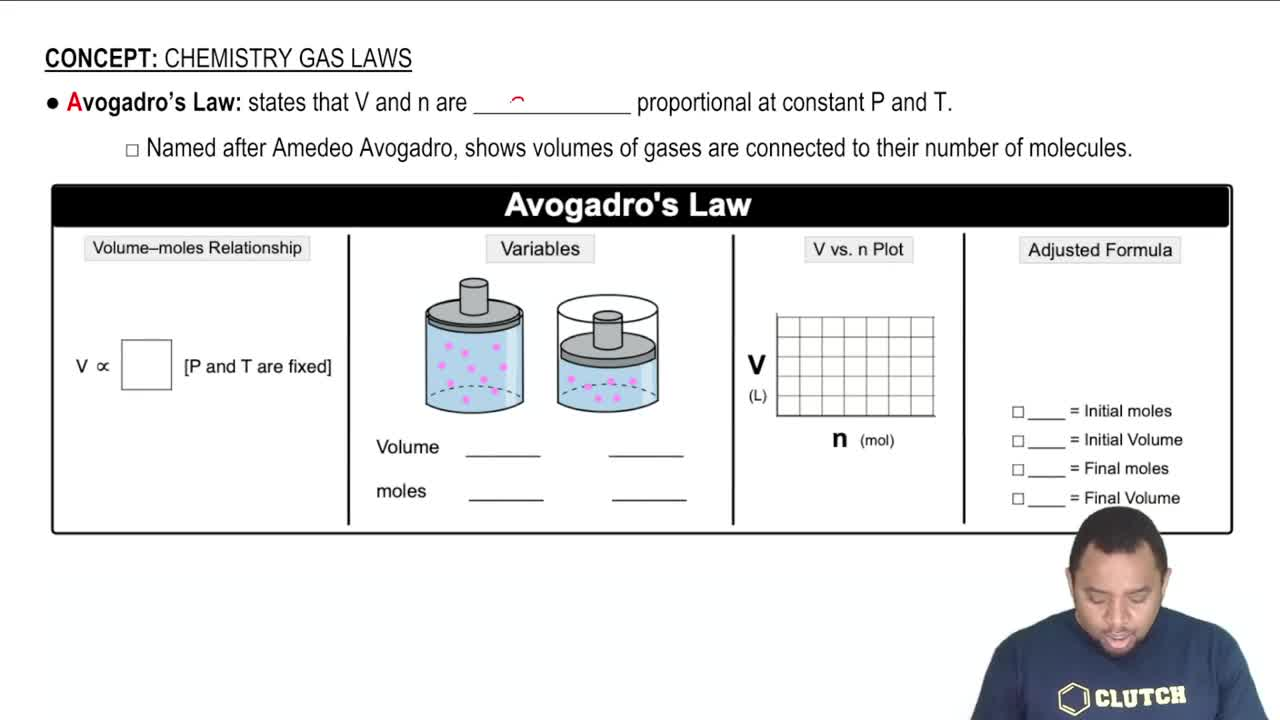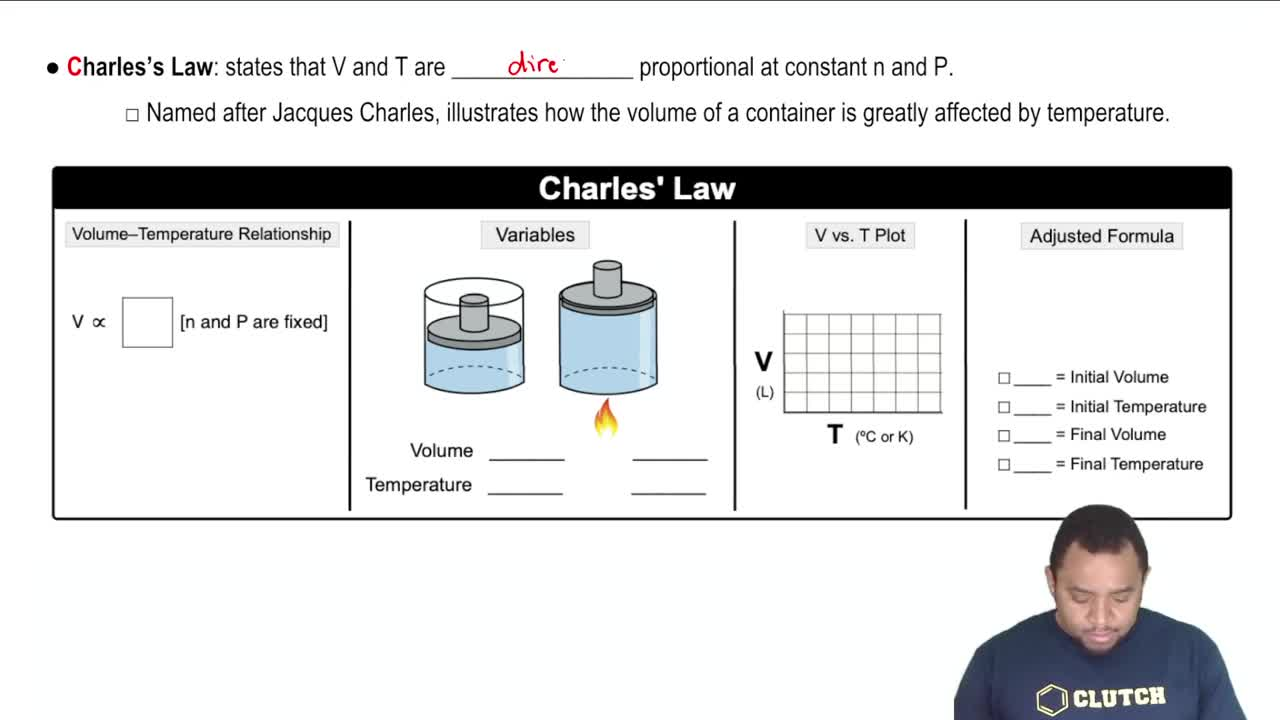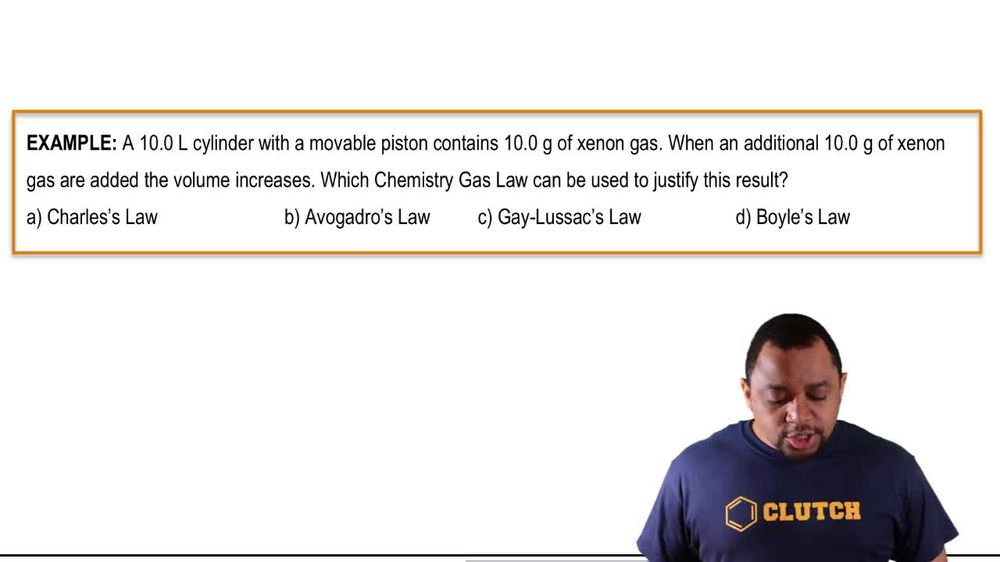Start typing, then use the up and down arrows to select an option from the list.
1. 7. Gases2. Chemistry Gas Laws# Chemistry Gas Laws

by Jules Bruno
120 views
1
1
Doyle's law states that volume and pressure are inversely proportional at constant moles and temperature. Now it's named after robert Boyle and it illustrates how the volume of a container is greatly affected by pressure changes. Now here, how do we depict this relationship? When we say they're inversely proportional, we can say that they're on different levels. So we're gonna say volume is inversely proportional to one over inversely proportional pressure. Which means that v the proportionality symbol one over P. This shows us an inverse relationship between volume and pressure. Think of it as volume being a numerator pressure, being a denominator there on different levels. So they are different from one another. If one goes up the other one has to go down. Now this is illustrated if we take a look at variables here, if we take a look, we have two containers with movable pistons. Volume is just the space within my container. So if we look at this image, we can say that the volume is pretty high pressure represents the downward force that we have on the piston. Now the downward force on the piston must be pretty low, which is why the piston hasn't slid down lower. Okay, and here we can see volume is high pressure is low. Now let's say that we garnered enough force from the pressure. We're able to push down on this piston. We can see that the volume now is smaller, so the volume now is low and that's a direct result of the pressure being hired. Now how do we depict this inverse relationship graphically Well here to show an inverse relationship between two variables, you would show it like this? So this graph that showed me that my volume is decreasing over time and as a result of pressure is increasing over time. This is how we depict an inverse relationship between two variables. Now, how do we show Boyle's law formula in in the in form of a digested formula. Here we say that it becomes P one V one equals P two, V two. This represents our adjusted formula. Also, our Boyle's Law formula Where P one is our initial volume. V one is our initial RP 1's our initial pressure. V one is our initial volume. P two is our final pressure and V two is our final volume. Now, remember we went over how we derived these different types of formulas under the ideal gas laws application section. If you don't know what that is or if you haven't seen those videos yet, I suggest you go back and take a look at how we can derive this formula. Now we just know that it's connected to Boyle's law and therefore called the Boyle's Law formula. Okay, so keep this in mind. Boyle's law says that pressure and volume are inversely proportional, meaning if one is high the other one would be low